"Calculate84" App Analytics: ASO Keyword Monitoring | ASOTools

# "Calculate84" App Analytics: ASO Keyword Monitoring | ASOTools

Are you doing Calculate84 app analytics for better app store ASO strategies? Whether Calculate84 in app store keep on top? What keyword tactics Calculate84 take to rank well in app store? Are app store keywords like "calculator 84", "statistics calculator", "stats calculator" matter in Calculate84 ranking?

Free ASO tools for Google Play & App Store, ASOTools will reveal the app store keywords secrets of Calculate84.

## App Analytics: Calculate84

Calculate84-Intended for use with TI-84, published by Rishav Mehta in app store, released on 2019-08-17, and last updated 2021-05-04. It belongs to Education category, and Education category is ranking 62 in app store. Currently, Calculate84 has 1261 ratings, the average rating is 4. Additionally, it had 60000 downloads and - revenue last month in app store worldwide, and the daily active users is -.

More Calculate84 info and other app analytics can be found freely in ASOTool.

## ASO Keyword Monitoring: Calculate84

### 1.Calculate84 Top 10 App Store Keywords

 Keyword Search Volume KD Related Apps Calculate84's Rank calculator 84 33 4661 49 238 1 statistics calculator 31 4640 12 209 1 stats calculator 19 4607 18 211 1 caculator 84 31 4605 24 53 1 ti 83 plus 5 4605 21 41 1 college calculator 6 4605 42 241 1 ti nspire 20 4605 16 58 1 lighting calculations lite 1 450 17 99 1 lighting calculations pro 1 425 17 99 1 calculate84 45 47 28 54 1

App store keywords matter for ASO. If your app is similar to Calculate84, when you optimize app store keyword ranking, pay attention to the metrics above. ASOTools will show you all of them for free.

Obviously, Calculate84 is ranking for "calculator 84", "statistics calculator", "stats calculator", "caculator 84", "ti 83 plus", "college calculator", "ti nspire", "lighting calculations lite", "lighting calculations pro", and "calculate84" these top 10 keywords in app store.

Also, you'll find Calculate84's position in app store search result. Calculate84 is ranking 1 for calculator 84, ranking 1 for statistics calculator, ranking 1 for stats calculator, and ranking 1 for caculator 84. For more Calculate84 keyword monitoring, please visit asotools.io.

### 2. Top 5 Keyword-Related Apps& App Analytics

• calculator 84  Related Apps & App Analytics
 Apps Average Star Ratings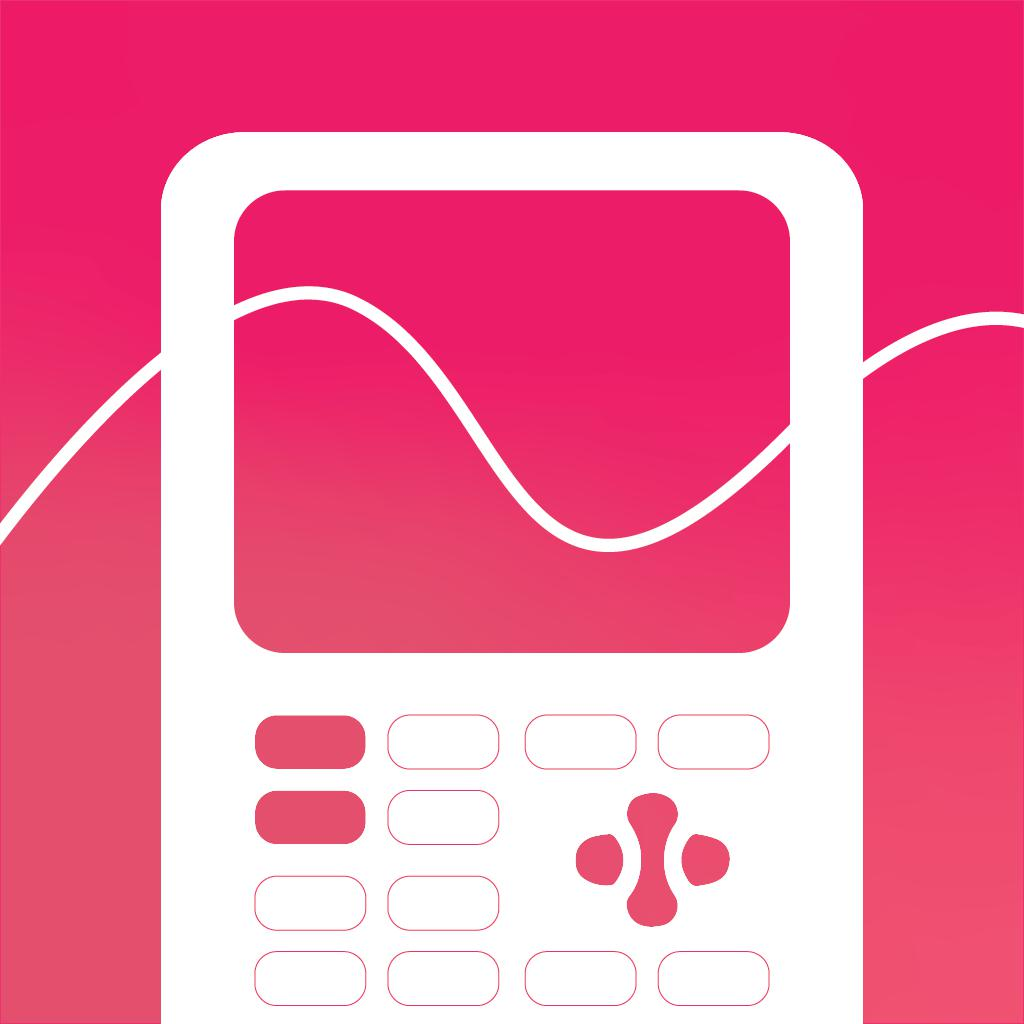Calculate84-Intended for use with TI-84 4 1261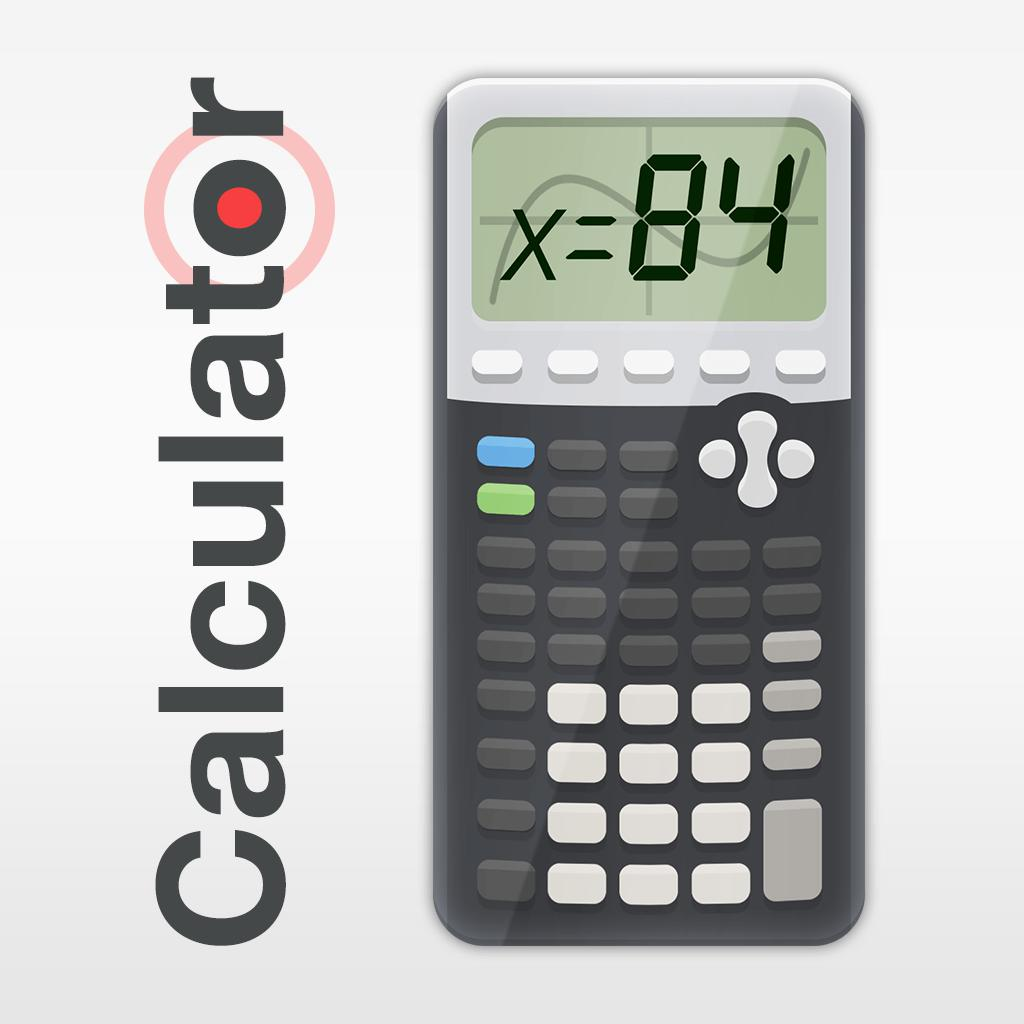Graphing Calculator X84-Designed for use with Ti-84 4 35200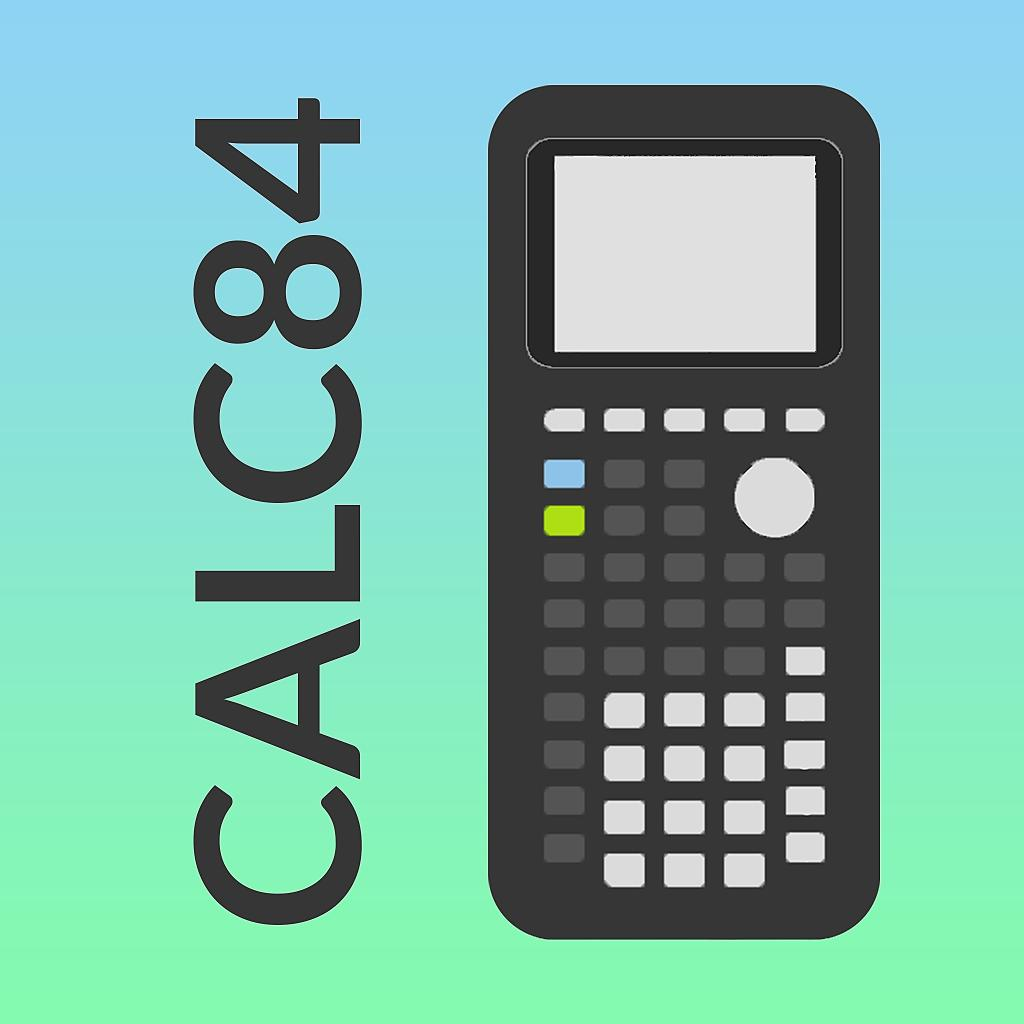Ncalc - Graphing Calculator 84-Designed for use with Ti-84 4 633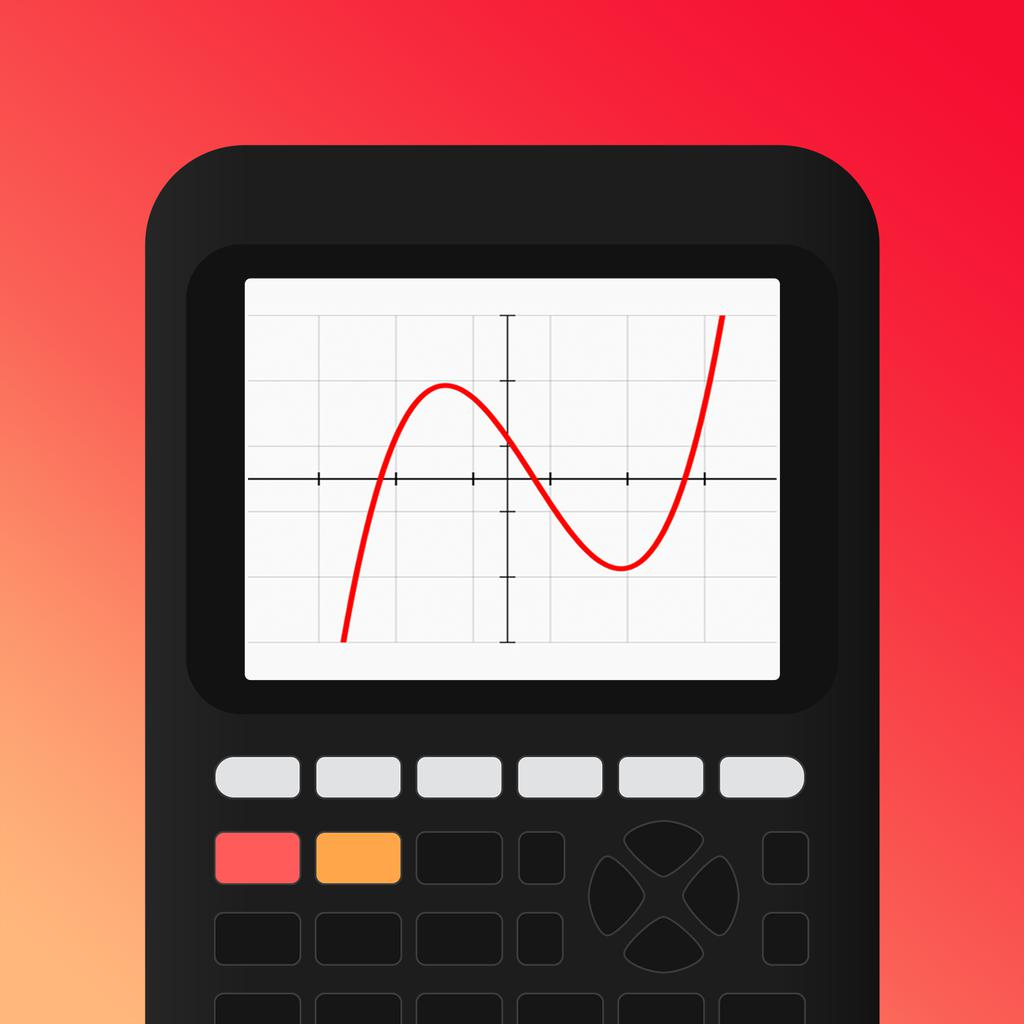Taculator Graphing Calculator-Statistics - Matrix - Graph 4 4264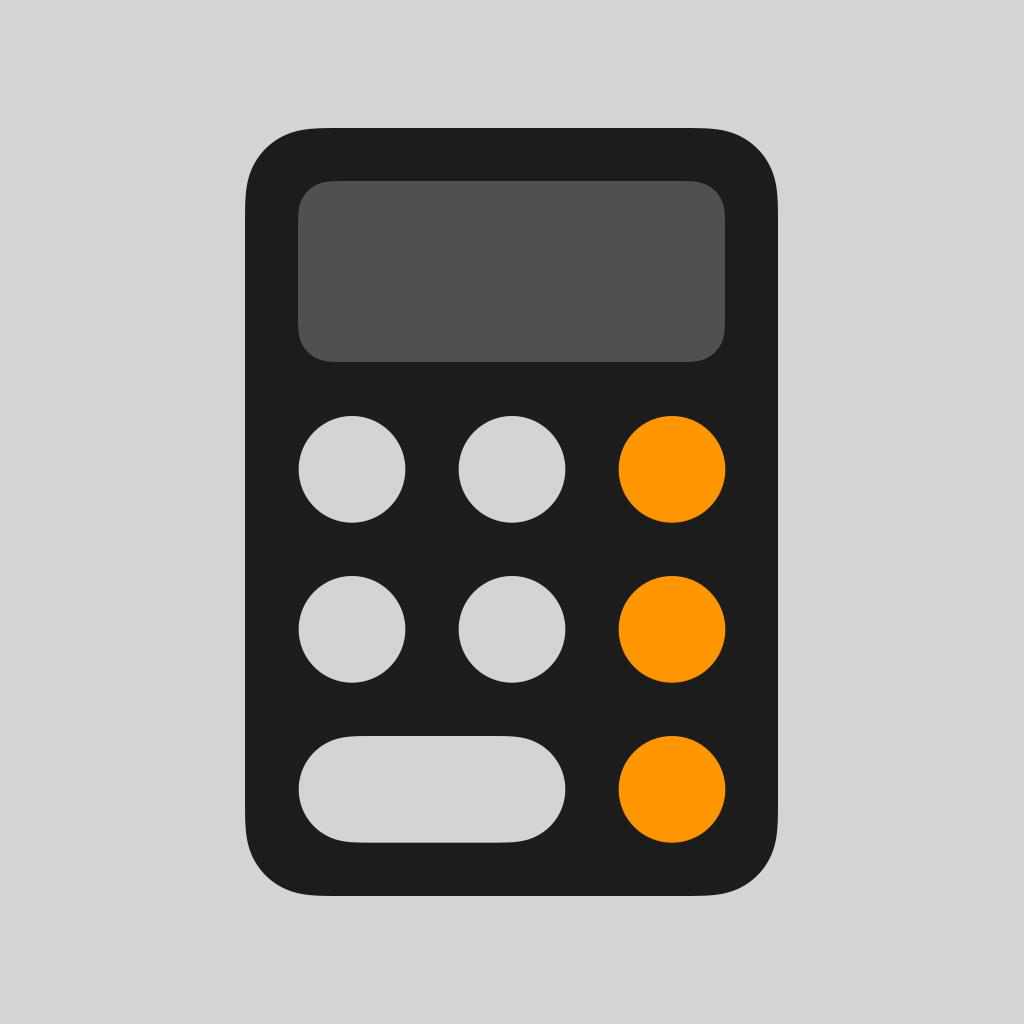Calculator - -

"calculator 84" has 238 apps. Top 5 are Calculate84, Graphing Calculator X84, Ncalc - Graphing Calculator 84, Taculator Graphing Calculator, and Calculator.

• statistics calculator  Related Apps & App Analytics
 Apps Average Star RatingsCalculate84-Intended for use with TI-84 4 1261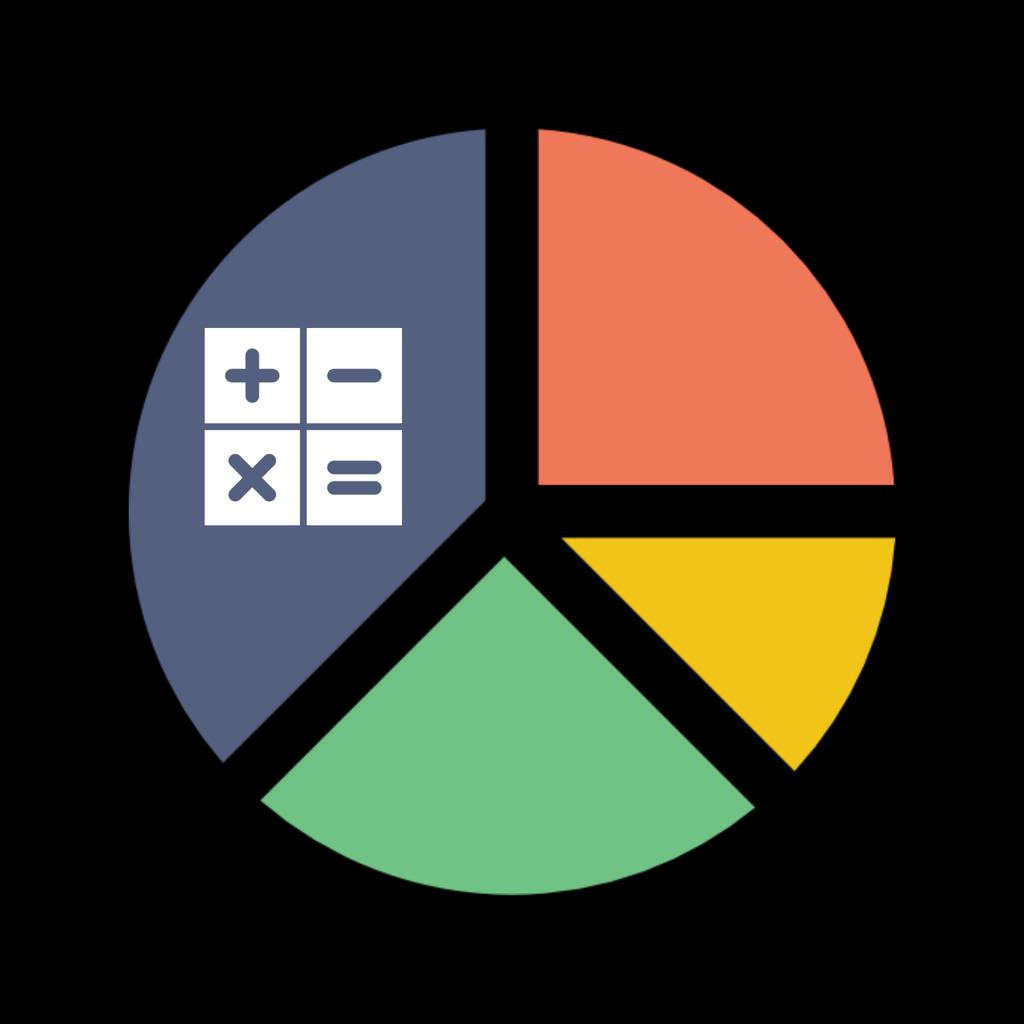Calculator Of Statistics-Statistics Calculator - -StatSuite (Statistics Suite)-Professional Statistics Suite 4 7Taculator Graphing Calculator-Statistics - Matrix - Graph 4 4264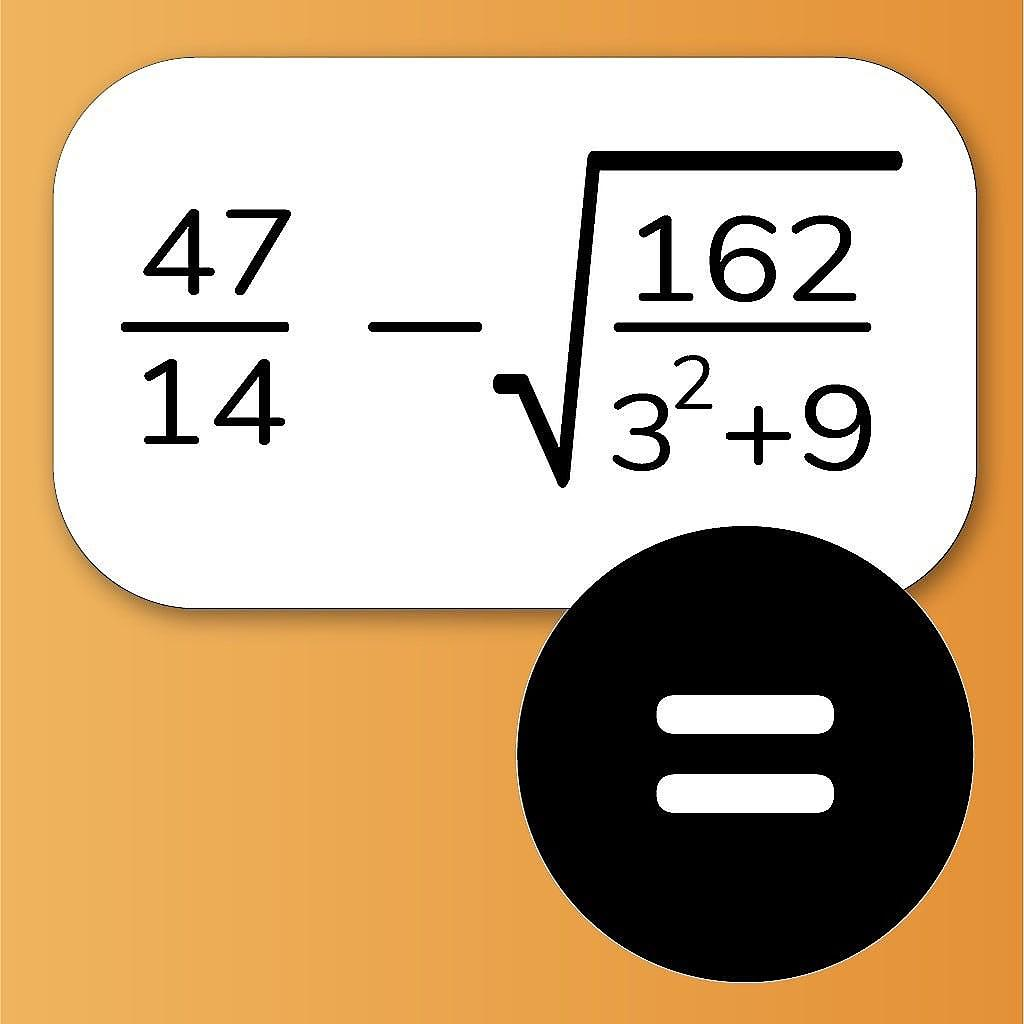NCalc Scientific Calculator +-Designed for math calculator 5 912

"statistics calculator" has 209 related apps in total. Top 5 are Calculate84, Calculator Of Statistics, StatSuite (Statistics Suite), Taculator Graphing Calculator, and NCalc Scientific Calculator +.

• stats calculator  Related Apps & App Analytics
 Apps Average Star RatingsCalculate84-Intended for use with TI-84 4 1261Microsoft Math Solver - HW app-Math Homework Scanner & Tutor 5 20565Graphing Calculator X84-Designed for use with Ti-84 4 35200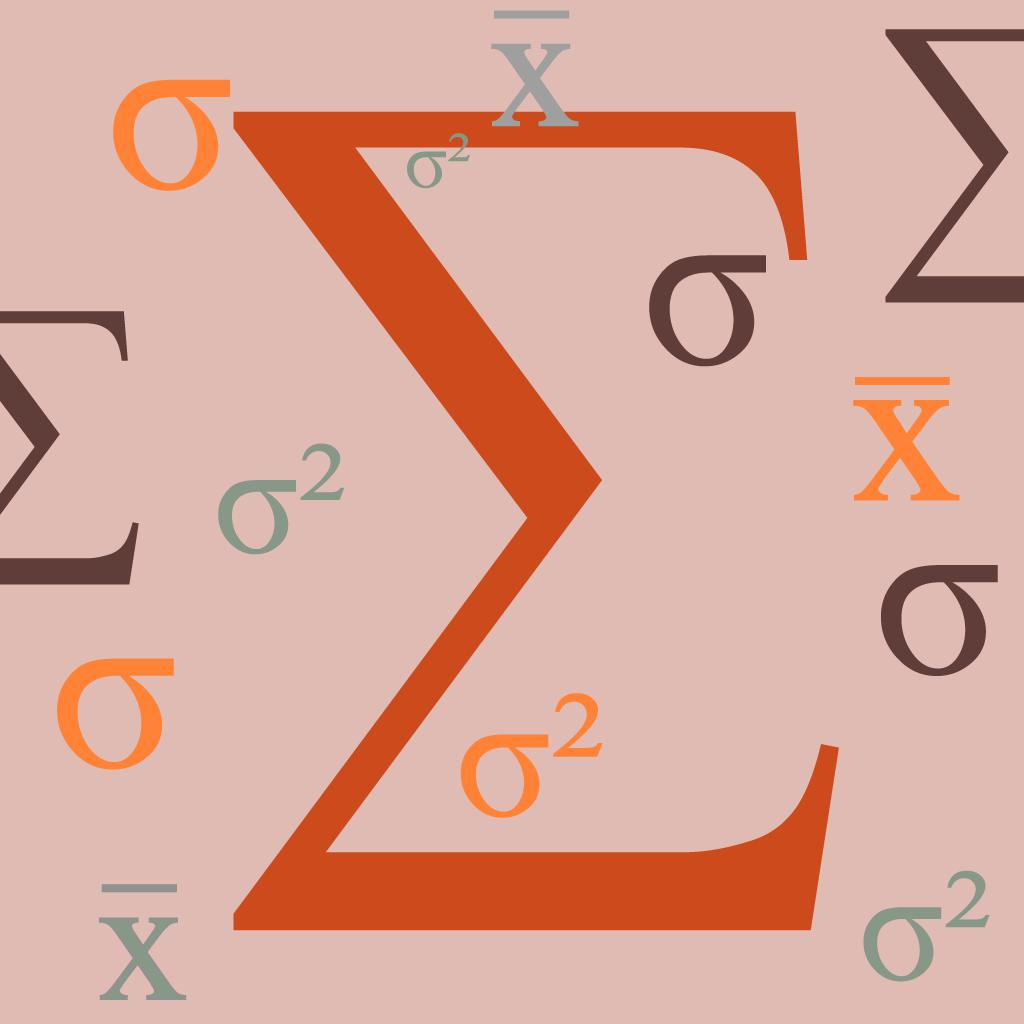Statistics Calculator Lite 2 16Mathway: Math Problem Solver-Homework scanner & calculator 5 309194

"stats calculator" has 211 related apps in app store. Top 5 are Calculate84, Microsoft Math Solver - HW app, Graphing Calculator X84, Statistics Calculator Lite, and Mathway: Math Problem Solver.

• caculator 84  Related Apps & App Analytics
 Apps Average Star RatingsCalculate84-Intended for use with TI-84 4 1261Graphing Calculator X84-Designed for use with Ti-84 4 35200Ncalc - Graphing Calculator 84-Designed for use with Ti-84 4 633Taculator Graphing Calculator-Statistics - Matrix - Graph 4 4264Calculator - -

"caculator 84" has 53 related apps. Top 5 apps are Calculate84, Graphing Calculator X84, Ncalc - Graphing Calculator 84, Taculator Graphing Calculator, and Calculator.

• ti 83 plus  Related Apps & App Analytics
 Apps Average Star RatingsCalculate84-Intended for use with TI-84 4 1261Graphing Calculator X84-Designed for use with Ti-84 4 35200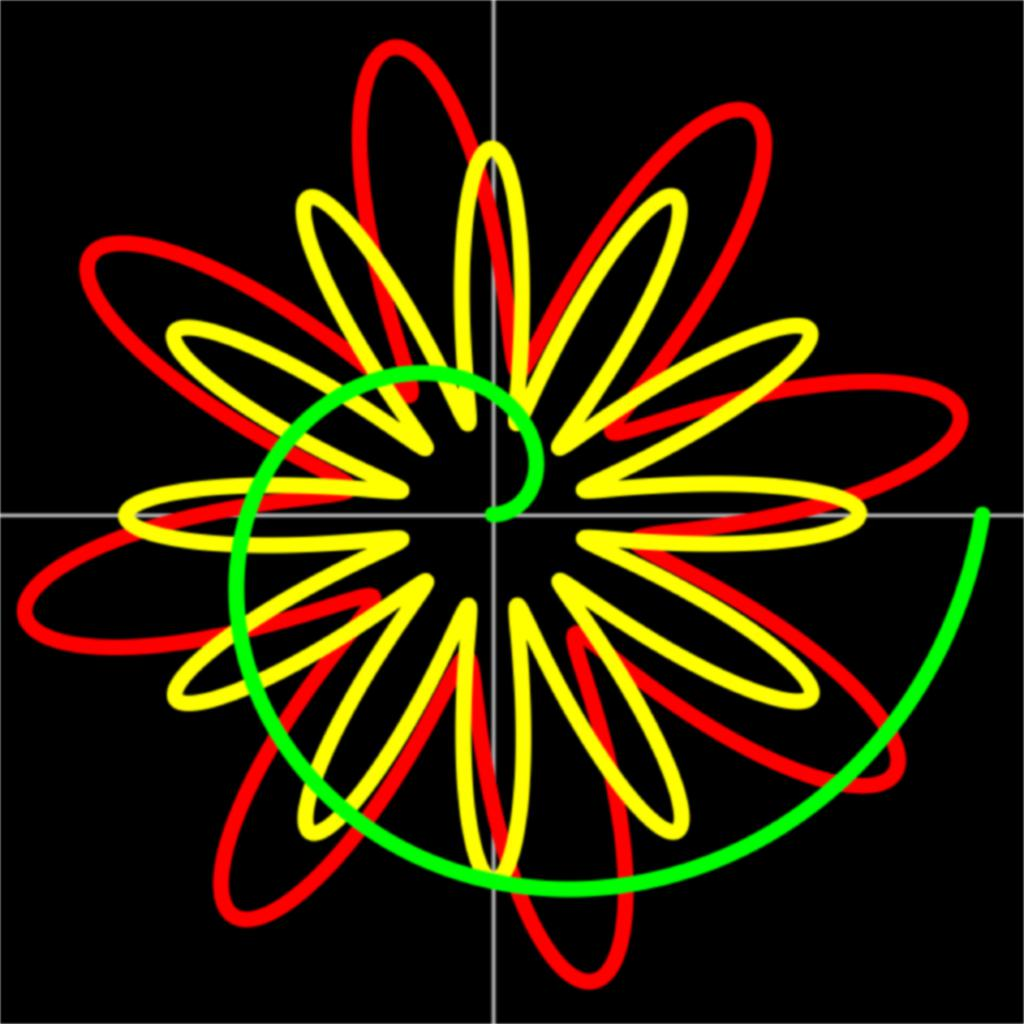GraphNCalc83-Graphing calculator 5 1497Taculator Graphing Calculator-Statistics - Matrix - Graph 4 4264Ncalc - Graphing Calculator 84-Designed for use with Ti-84 4 633

41 apps are ranking for "ti 83 plus" in app store. Top 5 apps are Calculate84, Graphing Calculator X84, GraphNCalc83, Taculator Graphing Calculator, and Ncalc - Graphing Calculator 84.

As you can see, Calculate84, Calculate84, and Graphing Calculator X84 are ranking for calculator 84, also, Calculate84, Calculate84, Calculator Of Statistics and Taculator Graphing Calculator are all competing the keyword statistics calculator.

Before promoting your apps in app store, you'd better perform app analytics for better ASO tactics, and ASOTools, free ASO tools will help you with App store keywords optimization.